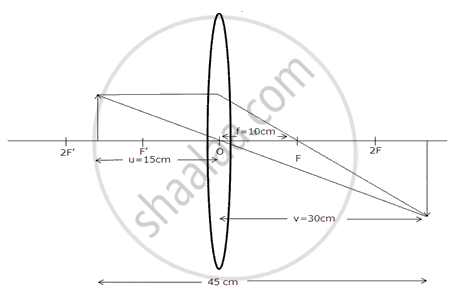Advertisement Remove all ads

# A Convex Lens Produces an Image on a Screen Twice the Size of the Object. the Distance Between the Object and Screen is 45 Cm. Find the Distance of the Lens from the Object and Its Focal Lengt - Physics

Diagram
A convex lens produces an image on a screen twice the size of the object. The distance between the object and screen is 45 cm. Find the distance of the lens from the object and its focal length by drawing a ray diagram.
Advertisement Remove all ads

#### SolutionMagnification, m = "I"/"O" = "u"/"v" = 2 (given)

⇒ v = 2 u

u + v = 45 cm (given)

u + 2u = 45cm

3u = 45cm

therefore u = 15 cm

Distance of lens from the object is 15 cm.

⇒ v = 2u = 30cm

We know, 1/"f" = 1/"u" + 1/"v"

= 1/15 + 1/30 = 3/10 = 1/10

⇒ f = 10 cm

Focal length is 10 cm.

Concept: Convex Lens
Is there an error in this question or solution?
Advertisement Remove all ads

#### APPEARS IN

Frank ICSE Class 10 Physics Part 2
Chapter 2 Light
Exercise 2.2 | Q 1 | Page 103
Advertisement Remove all ads
Advertisement Remove all ads
Share
Notifications

View all notifications

Forgot password?
Course